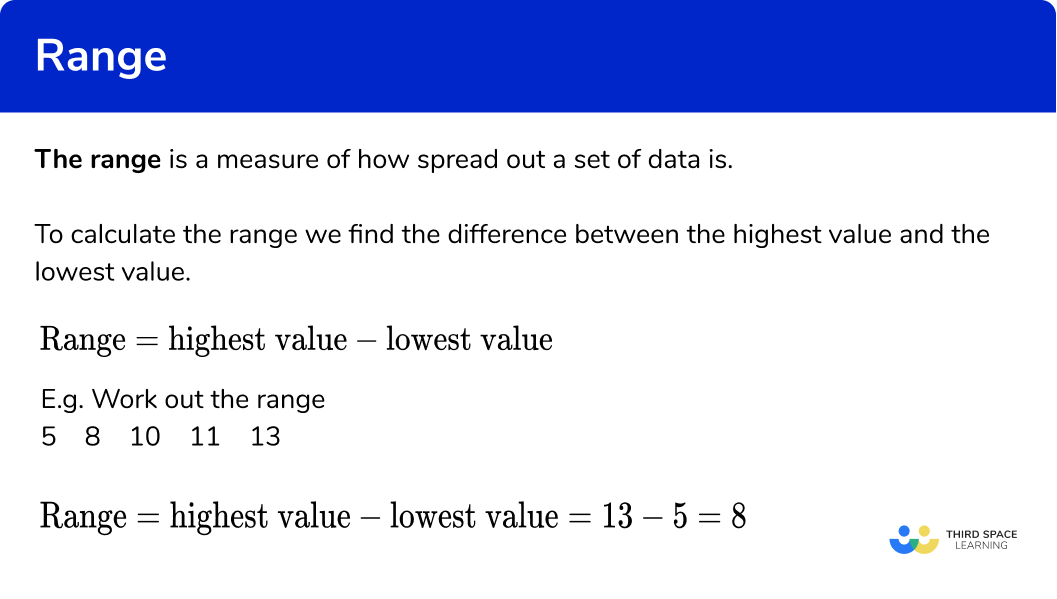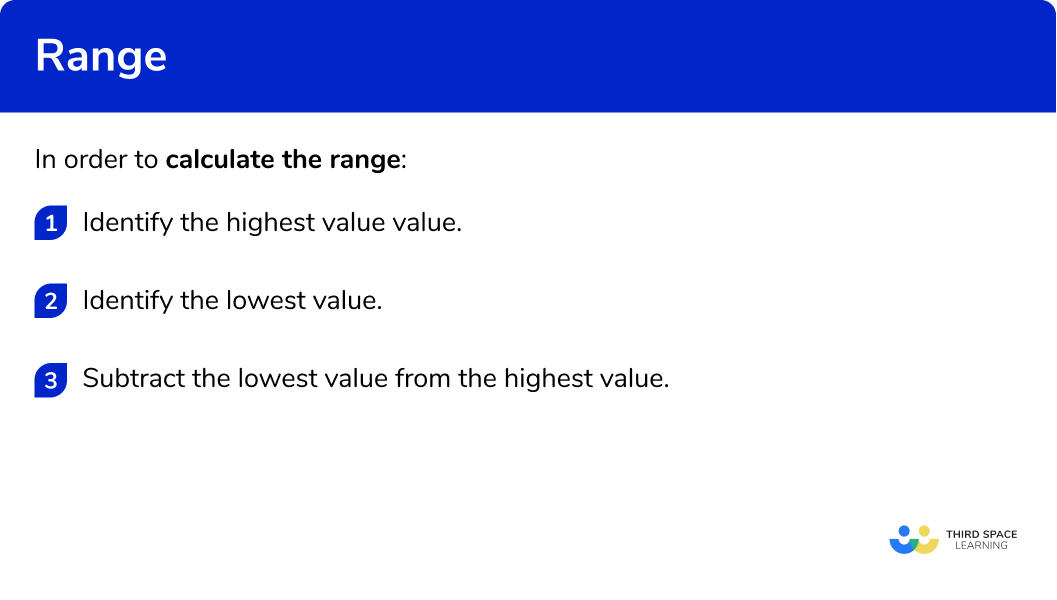# Range

Here we will learn about the range in maths, including what the range is and how to calculate it.  We will also learn how to solve problems involving the range.

There are also range worksheets based on Edexcel, AQA and OCR exam questions, along with further guidance on where to go next if you’re still stuck.

## What is range?

The range is a measure of how spread out a set of data is.

To calculate the range we find the difference between the highest value and the lowest value.  (The highest value is sometimes called the largest value or largest number.  The lowest value is sometimes referred to as the smallest value or smallest number).

\text{Range}=\text{highest value}-\text{lowest value}

For example, work out the range

\text{Range}=\text{highest value}-\text{lowest value}=13-5=8

### What is range?## Averages and range

The range is a type of statistic. Other statistics include the mean, the median and the mode. Each statistic allows us to analyse the data set, looking at: the spread of the data (the range), the location of the middle value (the median), the average value (the mean), and the most common value (the mode).

When we find the average for a set of data, we are referring to the mean average.

## How to calculate range

In order to calculate the range:

1. Identify the highest value.
2. Identify the lowest value.
3. Subtract the lowest value from the highest value.

### How to calculate range## Related lessons on mean, median, mode

Range is part of our series of lessons to support revision on mean, median, mode. You may find it helpful to start with the main mean, median, mode lesson for a summary of what to expect, or use the step by step guides below for further detail on individual topics. Other lessons in this series include:

## Range maths examples

### Example 1: finding the range for an ordered list of numbers

Work out the range of this set of numbers

1. Identify the highest value.

Look through the set of values and find the highest value (or highest value).

The highest value is 15 .

2Identify the lowest value.

Look through the set of values and find the lowest value (or lowest number).

The lowest value is 3.

3Subtract the lowest value from the highest value.

The range is the difference between the highest and lowest values.

\text{Range}=\text{highest value}-\text{lowest value}=15-3=12

The range is 12.

### Example 2: finding the range for an ordered list of numbers

Work out the range of this set of numbers

Identify the highest value.

Identify the lowest value.

Subtract the lowest value from the highest value.

## How to solve problems involving range

In order to use the range with problem solving:

1. Write down how to find the range.
2. Substitute the values we are given.
3. Work out the missing value.

## Problems involving range examples

### Example 3: finding the highest value

The range of a set of data is 12.

The lowest value is 25.

Work out the highest value.

Write down how to find the range.

Substitute the values we are given.

Work out the missing value.

### Example 4: finding the highest value

The range of a set of data is 14.

The lowest value is 38.

Work out the highest value.

Write down how to find the range.

Substitute the values we are given.

Work out the missing value.

### Example 5: finding the lowest value

The range of a set of data is 8.

The highest value is 14.

Work out the lowest value.

Write down how to find the range.

Substitute the values we are given.

Work out the missing value.

### Example 6: finding the lowest number

The range of a set of data is 17.

The highest value is 28.

Work out the lowest value.

Write down how to find the range.

Substitute the values we are given.

Work out the missing value.

### Common misconceptions

• Lowest and highest values

Sometimes the list of values is not in order of size.  Make sure that you are definitely using the lowest and highest values.

• Remember to calculate the range

Writing the range as lowest number to highest number with a dash in between is incorrect.  To calculate the range you need to find the difference between these values.

E.g. What is the range of this set of values?

The range is not 1-8 or 1- to -8

The range is 8-1=7

### Practice range maths questions

1. Work out the range of this set of data:

141398The range is calculated by finding the difference between the highest and lowest values.

\text{Range}=\text{highest value}-\text{lowest value}=11-3=8

2. Work out the range of this set of data:

29141328The range is calculated by finding the difference between the highest and lowest values.

\text{Range}=\text{highest value}-\text{lowest value}=21-8=13

3. The range of a set of data is 6. The lowest value is 5. What is the highest value?

10111213The highest value is found by adding the range on to the lowest value.

\text{Highest value}=\text{lowest value}+\text{Range}=5+6=11

4. The range of a set of data is 17. The lowest value is 14. What is the highest value?

343031The highest value is found by adding the range on to the lowest value.

\text{Highest value}=\text{lowest value}+\text{Range}=14+17=31

5. The range of a set of data is 9. The highest value is 17. What is the lowest value?

782627The highest value is found by subtracting the range from the highest value.

\text{Lowest value}=\text{highest value}-\text{Range}=17-9=8

6. The range of a set of data is 16. The highest value is 34. What is the lowest value?

17184950The highest value is found by subtracting the range from the highest value.

\text{Lowest value}=\text{highest value}-\text{Range}=34-16=18

### Range maths GCSE questions

1. Here is a list of numbers.

Work out the range of the numbers in the list.

(2 marks)

\text{Range}=\text{highest value}-\text{lowest value}=28-9

(1)

\text{Range}=19

(1)

2. Here is a list of numbers.

Work out the range of the numbers in the list.

(2 marks)

\text{Range}=\text{highest value}-\text{lowest value}=21-12

(1)

\text{Range}=9

(1)

2. Jack chooses four numbers.

The highest of these four numbers is 23.

The range of these four numbers is 17.

Find the lowest of these four numbers.

(2 marks)

\text{Lowest value}=\text{highest value}-\text{Range}=23-17

(1)

\text{Lowest value}=6

(1)

## Learning checklist

You have now learned how to:

• Work out the range
• Solve problems involving the range

## Still stuck?

Prepare your KS4 students for maths GCSEs success with Third Space Learning. Weekly online one to one GCSE maths revision lessons delivered by expert maths tutors.

Find out more about our GCSE maths tuition programme.